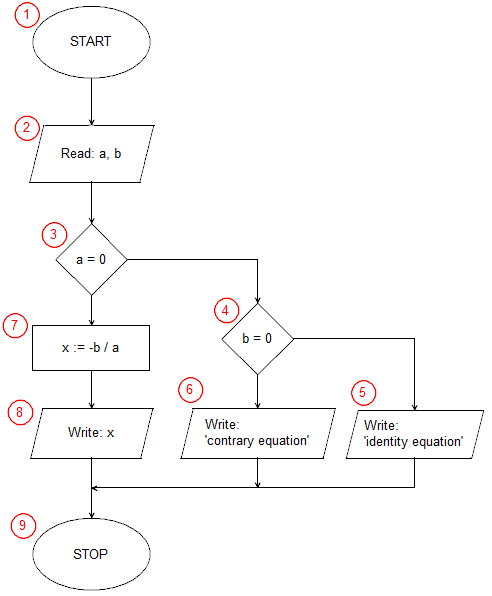# Implementation of a Linear equation solving algorithm in Javaimport java.util.Scanner; public class Linear{ public static void main(String[] args) { float a,b; Scanner key=new Scanner(System.in); System.out.print("a="); a=key.nextFloat(); System.out.print("b="); b=key.nextFloat(); if(a==0) if(b==0) System.out.println("identity equation"); else System.out.println("contrary equation"); else { float x; x=-b/a; System.out.print("x="); System.out.println(x); } } }

In the first line of the program, we inform you that we will use the Scanner class from the util java library. The Scanner class will be used to load input data in our program. In java, program execution starts with calling the public main() function contained in the class whose name matches the program name (the program must be saved in the Linear.java file and then compiled to the Line.class file). The definition of the Linear class starts in the 2nd program line. In our example, the main() function is the only function of the program. Line 3 contains the beginning of the main function definition, this is the beginning of the implementation of our algorithm (block START (1)). In the next line, we declare two variables a, b, which we will be using in a moment. In Java, variables can be declared anywhere before they are used, so we do not declare an x ​​variable here immediately, which we may not need at all. In 5 lines, we declare and initialize the key variable as a Scanner object. We indicate that the source of text data will be the System.in stream, or keyboard.

The lines 6 to 9 perform the input/output (2) operation in which the input data is taken - the coefficients a and b of the equation.

The conditional statement from line 10 is the selection block (3) from our algorithm. The instruction checks whether the coefficient a is equal to 0. When the condition is satisfied, the instructions included in lines 11 to 14 are implemented (in the algorithm block selection (4) and input-output blocks (5) and (6)). When the condition is not satisfied, the statement after the else keyword is executed, in our case it is a compound instruction, enclosed in logical brackets {} between lines 15 and 20. First, on line 16, we declare the variable x, now we know that we will needed, then on line 17 we calculate the solution of the equation x = -b / a (operational block (7)), then in lines 18 and 19 we write the result obtained (input-output block (8)).

The curly brace } from line 21 ends the main function and also the operation of the entire program (end block of the algorithm (9)), while the curly bracket in line 22 ends the definition of the Linear class.